# Smoothing and simplifying surfaces#

When working with surface meshes, it is a common task to simplify these meshes to enable their analysis. Also smoothing them might be necessary, for example to avoid measuring voxel-lated structures.

import napari_process_points_and_surfaces as nppas
import vedo


Our starting point is the simulated branchoid we saved to disk earlier in this chapter.

mesh = vedo.load("../../data/branchoid.ply")
surface = nppas.to_napari_surface_data(mesh)
surface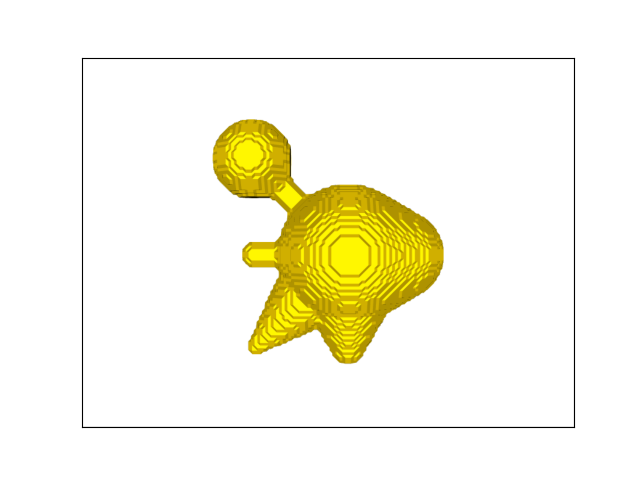nppas.SurfaceTuple
 origin (z/y/x) [0. 0. 0.] center of mass(z/y/x) 50.000,46.575,42.589 scale(z/y/x) 1.000,1.000,1.000 bounds (z/y/x) 25.500...74.5002.500...88.5002.500...83.500 average size 31.277 number of vertices 19040 number of faces 38076

## Smoothing meshes#

The circular structures you see in this dataset result from the origin of the data: The surface was created from a binary image. The lines correspond to voxel edges. Measuring the surface area would be misleading. Thus, we need to smooth the dataset before doing quantitative measurements.

smoothed_surface = nppas.smooth_surface(surface)
smoothed_surface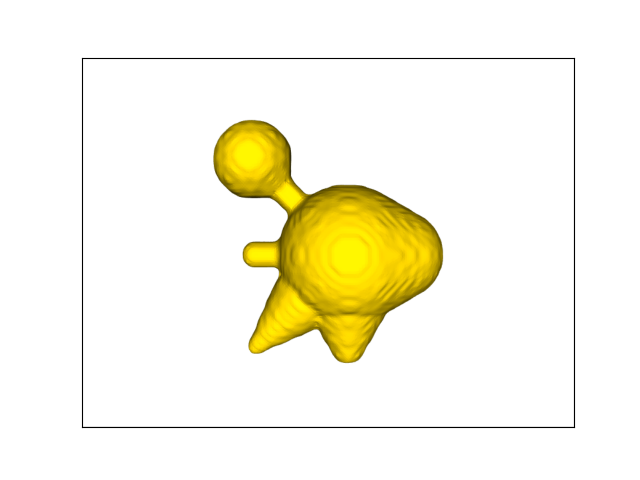nppas.SurfaceTuple
 origin (z/y/x) [0. 0. 0.] center of mass(z/y/x) 50.000,46.578,42.588 scale(z/y/x) 1.000,1.000,1.000 bounds (z/y/x) 25.347...74.6532.328...88.7042.334...83.678 average size 31.345 number of vertices 19040 number of faces 38076

We can also modify how fine the smoothing is applied.

smoothed_surface2 = nppas.smooth_surface(surface, pass_band=0.01)
smoothed_surface2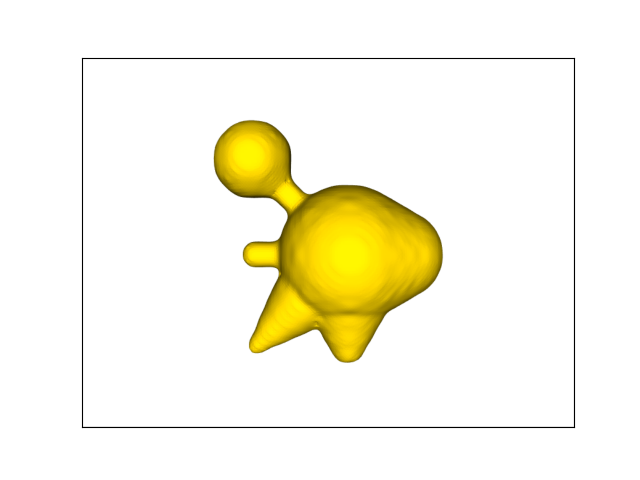nppas.SurfaceTuple
 origin (z/y/x) [0. 0. 0.] center of mass(z/y/x) 50.000,46.577,42.588 scale(z/y/x) 1.000,1.000,1.000 bounds (z/y/x) 25.360...74.6402.296...88.7972.302...83.693 average size 31.349 number of vertices 19040 number of faces 38076

The parameters also allow to remove local structure, in particular the number of iterations.

smoothed_surface3 = nppas.smooth_surface(surface, number_of_iterations=100,
pass_band=0.00001,
)
smoothed_surface3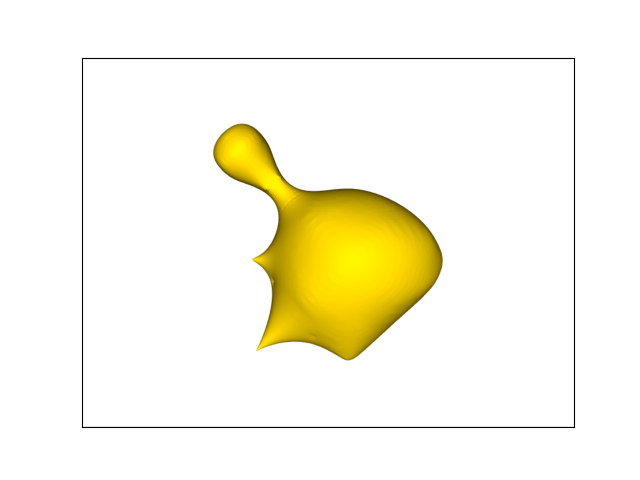nppas.SurfaceTuple
 origin (z/y/x) [0. 0. 0.] center of mass(z/y/x) 50.013,46.548,42.623 scale(z/y/x) 1.000,1.000,1.000 bounds (z/y/x) 27.575...72.3736.941...82.1927.009...79.894 average size 29.331 number of vertices 19040 number of faces 38076

There are also more functions for smoothing surfaces, e.g. based on moving least squares.

nppas.smooth_surface_moving_least_squares_2d(surface, smoothing_factor=0.2)

 ━━━━━━━━━━━━━━━━━━━━━━ elapsed: 5s (3714.7 it/s)             (3707.5 it/s)         working ...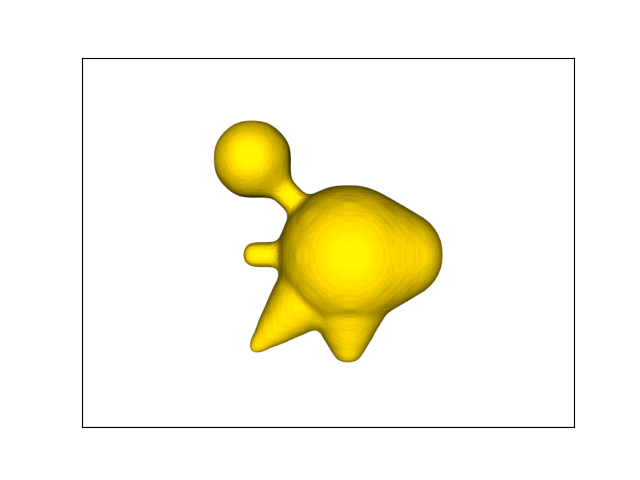nppas.SurfaceTuple
 origin (z/y/x) [0. 0. 0.] center of mass(z/y/x) 50.000,46.575,42.589 scale(z/y/x) 1.000,1.000,1.000 bounds (z/y/x) 25.500...74.5002.500...88.4882.500...83.500 average size 31.218 number of vertices 19040 number of faces 38076
nppas.smooth_surface_moving_least_squares_2d_radius(surface, smoothing_factor=0.2, radius=3)

 ━━━━━━━━━━━━━━━━━━━━━━ elapsed: 5s (3572.6 it/s)             (3577.5 it/s)         working ...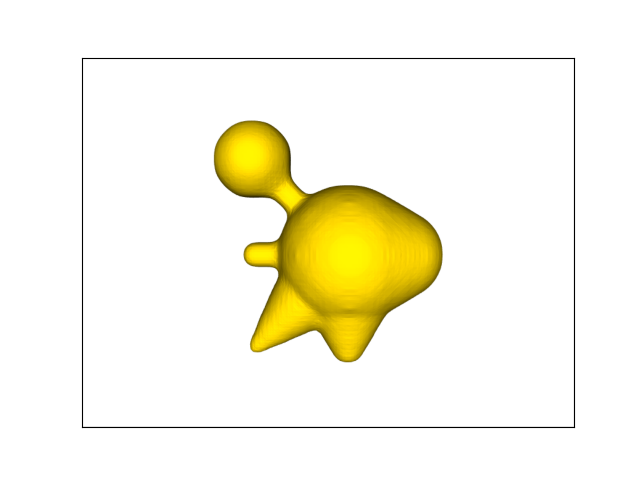nppas.SurfaceTuple
 origin (z/y/x) [0. 0. 0.] center of mass(z/y/x) 50.000,46.575,42.589 scale(z/y/x) 1.000,1.000,1.000 bounds (z/y/x) 25.500...74.5002.500...88.5002.500...83.500 average size 31.211 number of vertices 19040 number of faces 38076

## Simplifying surfaces meshes#

In case a surface mesh has too many vertices and faces, processing it may take long time. Too detailed surfaces mesh may not bring additional information as well. Thus, it might make sense to simplify surfaces, for example by reducing the number of vertices and faces by half.

simplified_surface = nppas.decimate_pro(smoothed_surface, fraction=0.5)
simplified_surface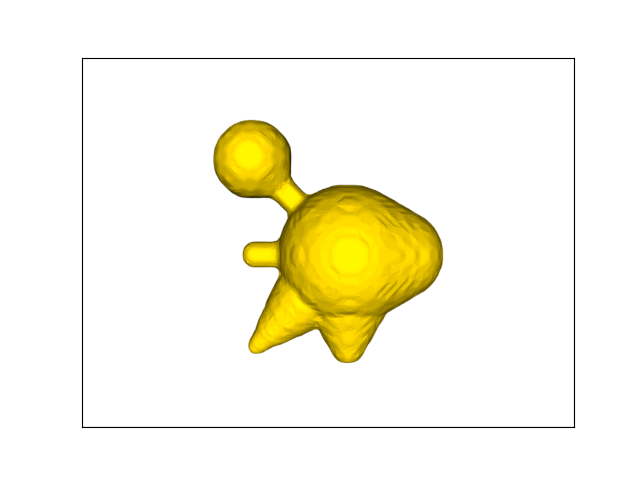nppas.SurfaceTuple
 origin (z/y/x) [0. 0. 0.] center of mass(z/y/x) 50.024,46.096,39.540 scale(z/y/x) 1.000,1.000,1.000 bounds (z/y/x) 25.347...74.6532.332...88.7042.338...83.678 average size 32.057 number of vertices 9521 number of faces 19038

If we simplify the surface too much, we may loose spatial information and resolution. The object may then not be represented appropriately anymore.

simplified_surface2 = nppas.decimate_quadric(smoothed_surface, fraction=0.1)
simplified_surface2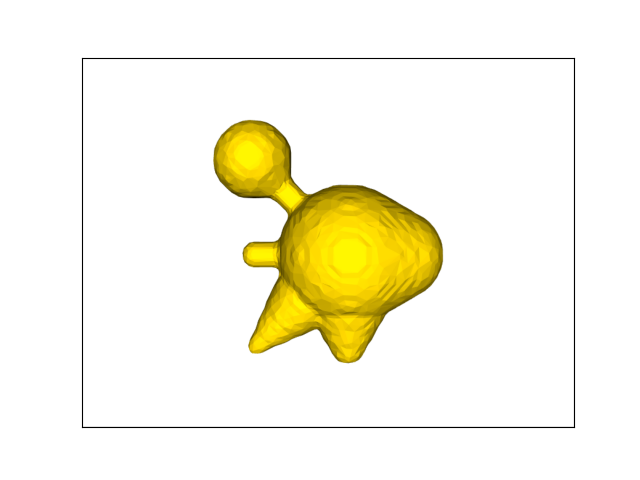nppas.SurfaceTuple
 origin (z/y/x) [0. 0. 0.] center of mass(z/y/x) 49.963,47.454,39.244 scale(z/y/x) 1.000,1.000,1.000 bounds (z/y/x) 25.366...74.6342.312...88.6782.318...83.672 average size 31.565 number of vertices 1905 number of faces 3806
simplified_surface2 = nppas.decimate_quadric(smoothed_surface, fraction=0.01)
simplified_surface2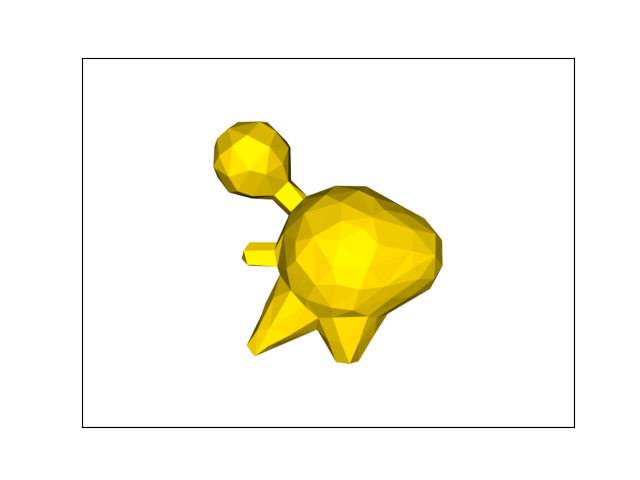nppas.SurfaceTuple
 origin (z/y/x) [0. 0. 0.] center of mass(z/y/x) 50.146,45.715,39.357 scale(z/y/x) 1.000,1.000,1.000 bounds (z/y/x) 25.440...74.6232.344...88.2582.412...83.760 average size 31.898 number of vertices 192 number of faces 380
simplified_surface2 = nppas.decimate_quadric(smoothed_surface, fraction=0.001)
simplified_surface2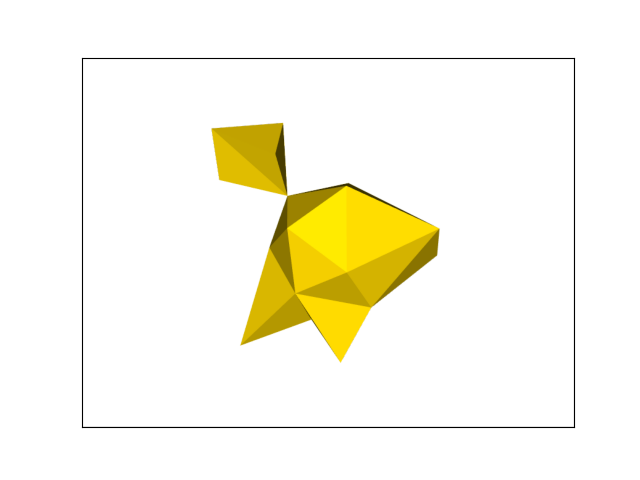nppas.SurfaceTuple
 origin (z/y/x) [0. 0. 0.] center of mass(z/y/x) 49.941,45.866,38.671 scale(z/y/x) 1.000,1.000,1.000 bounds (z/y/x) 25.548...75.9053.858...93.1073.380...86.408 average size 33.976 number of vertices 22 number of faces 38